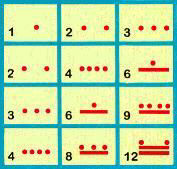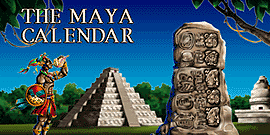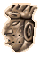THE MAYA CALCULATION ASSISTANT

The Maya Calculation Assistant published by The Maya World Studies Center (Centro de Estudios del Mundo Maya), is a calculator (matrix table) used by the Maya in their mathematical operations which also teaches the use of the Maya numerical system. As centuries ago, again today the Maya Calculation Assistant's main purpose is to ease the executing of mathematical calculations such as multiplication, squaring, division and finding a square root. Similar to the abacus of other cultures, it is a tool used by the ancient Maya to simplify and speed up daily counting.The Maya Calculation Assistant color posterClose up of the poster's cells

Using the Maya Calculation Assistant

Since the Maya numerical system is vigesimal, The Maya Calculation Assistant table contains 400 individual cells (20 x 20) each with its corresponding Maya numeral. Arabic numbers are also placed in each cell to simplify learning. To ease understanding, this table grows from top to bottom and from left to right, opposite to the Maya original.

When using the Maya Calculation table, please remember:

a) The top row and the first column on the left are the main row and column, they are numbered 1- 20.
b) When Maya numbers are written vertically (in any vigesimal position) dots always go on top of bars.
c) When there are dots, bars or a zero on top of dots, bars or a zero but separated by a wider space, they belong to a higher vigesimal position, so they have to be multiplied by the value of that position.
Please refer to the text of THE MAYA MATHEMATICAL SYSTEM for these positional values.

Multiplication

Numbers from 2 to 20 of the main row can be multiplied by any other from 2 to 20 on the main column, the result is easily found at the intersecting cell of the two numbers multiplied.

Squares

The first power of any number from 2 to 20 can be obtained in the same manner by multiplying any one of these main row numbers by the same number in the main column, the result is also in the intersecting cell of the two numbers multiplied.

Division

Any number from 4 to 400 located in the table's cells (dividend), except in the main row and column, can be divided by a number in the main row (divisor). The result is found in the corresponding cell of the main column. This is also true if the divisor is in the main column instead, in which case the answer is found in the corresponding cell of the main row.

Square root

The square root of numbers from 4 to 400 located in cells of the diagonal row (that goes from 1 to 400) of the table, can be found in the corresponding cell of the main row or column.

The Maya World Studies Center asks for a contribution for the Maya Calculation Assisant poster, plus S&H.

 Click to view more Maya publicationsCentro de Estudios del Mundo Maya C. 53 # 319 x 42 y 44    V. la Hda. Mérida, Yucatán, México 97119 Email: contact@mayacalendar.com Maya World Studies Center http://mayacalendar.comHome